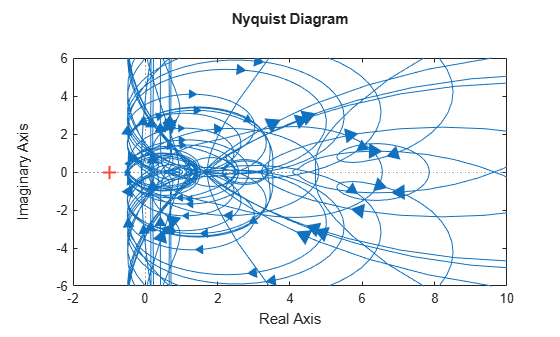Documentation

## Uncertain LTI Dynamics Elements

Uncertain linear, time-invariant objects, `ultidyn`, are used to represent unknown linear, time-invariant dynamic objects, whose only known attributes are bounds on their frequency response.

### Create Uncertain LTI Dynamics

You can create a 1-by-1 (scalar) positive-real uncertain linear dynamics element, whose frequency response always has real part greater than -0.5. Set the `SampleStateDimension` property to 5. Plot a Nyquist plot of 30 instances of the element.

```g = ultidyn('g',[1 1],'Type','Positivereal','Bound',-0.5); g.SampleStateDimension = 5;```
```nyquist(usample(g,30)) xlim([-2 10]) ylim([-6 6]);```### Properties of ultidyn Elements

Uncertain linear, time-invariant objects have an internal name (the `Name` property), and are created by specifying their size (number of outputs and number of inputs).

The property `Type` specifies whether the known attributes about the frequency response are related to gain or phase. The property `Type` may be `'GainBounded'` or `'PositiveReal'`. The default value is `'GainBounded'`.

The property `Bound` is a single number, which along with `Type`, completely specifies what is known about the uncertain frequency response. Specifically, if Δ is an `ultidyn` element, and if γ denotes the value of the `Bound` property, then the element represents the set of all stable, linear, time-invariant systems whose frequency response satisfies certain conditions:

If `Type` is `'GainBounded'`, $\stackrel{˙}{\overline{\sigma }}\left[\Delta \left(\omega \right)\right]\le \gamma$ for all frequencies. When `Type` is `'GainBounded'`, the default value for `Bound` (i.e., γ) is 1. The `NominalValue` of Δ is always the 0-matrix.

If `Type` is `'PositiveReal'`, Δ(ω) + Δ*(ω) ≥ 2γ· for all frequencies. When `Type` is `'PositiveReal'`, the default value for `Bound` (i.e., γ) is 0. The `NominalValue` is always (γ + 1 +2|γ|)I.

All properties of a `ultidyn` are can be accessed with `get` and `set` (although the `NominalValue` is determined from `Type` and `Bound`, and not accessible with `set`). The properties are

Properties

Meaning

Class

`Name`

Internal Name

`char`

`NominalValue`

Nominal value of element

`See above`

`Type`

`'GainBounded'```` |````'PositiveReal'`

`char`

`Bound`

Norm bound or minimum real

`scalar double`

`SampleStateDimension`

State-space dimension of random samples of this uncertain element

`scalar double`

`SampleMaxFrequency`

Maximum natural frequency for random sampling

`scalar double`

`AutoSimplify`

`'off'```` | {````'basic'````} |````'full'`

`char`

The `SampleStateDim` property specifies the state dimension of random samples of the element when using `usample`. The default value is 1. The `AutoSimplify` property serves the same function as in the uncertain real parameter.

### Time Domain of ultidyn Elements

On its own, every `ultidyn` element is interpreted as a continuous-time, system with uncertain behavior, quantified by bounds (gain or real-part) on its frequency response. However, when a `ultidyn` element is an uncertain element of an uncertain state space model (`uss`), then the time-domain characteristic of the element is determined from the time-domain characteristic of the system. The bounds (gain-bounded or positivity) apply to the frequency-response of the element. This is explained and demonstrated in .

### Interpreting Uncertainty in Discrete Time

The interpretation of a `ultidyn` element as a continuous-time or discrete-time system depends on the nature of the uncertain system (`uss`) within which it is an uncertain element.

For example, create a scalar `ultidyn` object. Then, create two 1-input, 1-output uss objects using the `ultidyn` object as their “D” matrix. In one case, create without specifying sample-time, which indicates continuous time. In the second case, force discrete-time, with a sample time of 0.42.

```delta = ultidyn('delta',[1 1]); sys1 = uss([],[],[],delta) USS: 0 States, 1 Output, 1 Input, Continuous System delta: 1x1 LTI, max. gain = 1, 1 occurrence sys2 = uss([],[],[],delta,0.42) USS: 0 States, 1 Output, 1 Input, Discrete System, Ts = 0.42 delta: 1x1 LTI, max. gain = 1, 1 occurrence ```

Next, get a random sample of each system. When obtaining random samples using `usample`, the values of the elements used in the sample are returned in the 2nd argument from `usample` as a structure.

```[sys1s,d1v] = usample(sys1); [sys2s,d2v] = usample(sys2); ```

Look at `d1v.delta.Ts` and `d2v.delta.Ts`. In the first case, since `sys1` is continuous-time, the system `d1v.delta` is continuous-time. In the second case, since `sys2` is discrete-time, with sample time 0.42, the system `d2v.delta` is discrete-time, with sample time 0.42.

```d1v.delta.Ts ans = 0 d2v.delta.Ts ans = 0.4200 ```

Finally, in the case of a discrete-time `uss` object, it is not the case that `ultidyn` objects are interpreted as continuous-time uncertainty in feedback with sampled-data systems. This very interesting hybrid theory is beyond the scope of the toolbox.